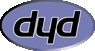# imagerotate

(PHP 4 >= 4.3.0, PHP 5, PHP 7)

imagerotateRotate an image with a given angle

### Description

resource imagerotate ( resource `\$image` , float `\$angle` , int `\$bgd_color` [, int `\$ignore_transparent` = 0 ] )

Rotates the `image` image using the given `angle` in degrees.

The center of rotation is the center of the image, and the rotated image may have different dimensions than the original image.

### Parameters

``` image```

An image resource, returned by one of the image creation functions, such as imagecreatetruecolor().

`angle`

Rotation angle, in degrees. The rotation angle is interpreted as the number of degrees to rotate the image anticlockwise.

`bgd_color`

Specifies the color of the uncovered zone after the rotation

`ignore_transparent`

If set and non-zero, transparent colors are ignored (otherwise kept).

### Return Values

Returns an image resource for the rotated image, or `FALSE` on failure.

### Changelog

Version Description
5.5.0 The `ignore_transparent` is now silently ignored.
5.5.0 This function is now affected by the interpolation method set by the imagesetinterpolation() function.
5.1.0 `ignore_transparent` was added.

### Examples

Example #1 Rotate an image 180 degrees

This example rotates an image 180 degrees - upside down.

``` <?php// File and rotation\$filename = 'test.jpg';\$degrees = 180;// Content typeheader('Content-type: image/jpeg');// Load\$source = imagecreatefromjpeg(\$filename);// Rotate\$rotate = imagerotate(\$source, \$degrees, 0);// Outputimagejpeg(\$rotate);// Free the memoryimagedestroy(\$source);imagedestroy(\$rotate);?> ```

The above example will output something similar to:### Notes

Note:

This function is affected by the interpolation method set by imagesetinterpolation().